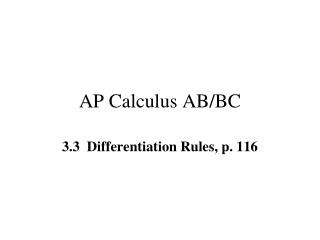DownloadDownload PresentationAP Calculus AB/BC

# AP Calculus AB/BC

Download Presentation## AP Calculus AB/BC

- - - - - - - - - - - - - - - - - - - - - - - - - - - E N D - - - - - - - - - - - - - - - - - - - - - - - - - - -
##### Presentation Transcript

1. Photo by Vickie Kelly, 2003 Greg Kelly, Hanford High School, Richland, Washington AP Calculus AB/BC 3.3 Differentiation Rules, p. 116 Colorado National Monument

2. The derivative of a constant is zero. If the derivative of a function is its slope, then for a constant function, the derivative must be zero. Constant Rule, p. 116 Example 1:

3. Power Rule, p. 116 This is part of a pattern. Examples 2a & 2b: The Power Rule works for both positive and negative powers

4. Constant Multiple Rule, p. 117 Example 3 where c is a constant and u is a differentiable function of x.

5. Constant Multiple Rule, p. 117 where c is a constant and u is a differentiable function of x. Sum and Difference Rules p. 117 where u and v are differentiable functions of x. Example 4a Example 4b (Each term is treated separately)

6. Horizontal tangents occur when slope = zero. Example 5 Find the horizontal tangents of: Plugging the x values into the original equation, we get: (The function is even, so we only get two horizontal tangents.)

7. Example 5 (cont.)

8. Example 5 (cont.)

9. Example 5 (cont.)

10. Example 5 (cont.)

11. Example 5 (cont.)

12. First derivative (slope) is zero at: Example 5 (cont.)

13. Product Rule, p. 119 Notice that this is not just the product of two derivatives. This is sometimes memorized as: Example 6:

14. Quotient Rule, p. 120 or Example 7: u′ u v v′ v2

15. Example 8 Find the tangent’s to Newton’s Serpentine at the origin and at the point (1, 2).

16. Example 8 (cont.) For the point (1, 2), plug in 1 for x. Next, find the slopes at the given points. For the point (0, 0), plug in 0 for x. y′ will be the slope. So, m = 0 and the equation for the tangent is y = 2 since the m is 0. So, m = 4 and the equation for the tangent is y = 4x since the y-intercept is 0.

17. Example 9: Find the first four derivatives of

18. is the first derivative of y with respect to x. is the second derivative. is the third derivative. is the fourth derivative. Higher Order Derivatives, p.122 (y double prime)